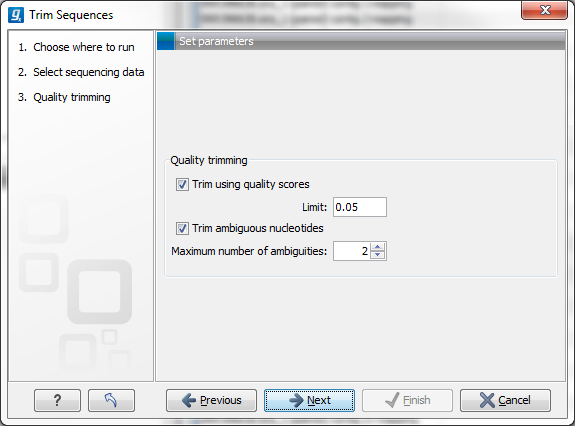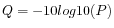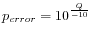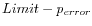## Quality trimming

This opens the dialog displayed in figure 23.1 where you can specify parameters for quality trimming.Figure 23.1: Specifying quality trimming.

The following parameters can be adjusted in the dialog:

• Trim using quality scores. If the sequence files contain quality scores from a base-caller algorithm this information can be used for trimming sequence ends. The program uses the modified-Mott trimming algorithm for this purpose (Richard Mott, personal communication):

Quality scores in the Workbench are on a Phred scale, and formats using other scales will be converted during import. The Phred quality scores (Q), defined as:, where P is the base-calling error probability, can then be used to calculate the error probabilities, which in turn can be used to set the limit for, which bases should be trimmed.

Hence, the first step in the trim process is to convert the quality score (Q) to an error probability:. (This now means that low values are high quality bases.)

Next, for every base a new value is calculated:. This value will be negative for low quality bases, where the error probability is high.

For every base, the Workbench calculates the running sum of this value. If the sum drops below zero, it is set to zero. The part of the sequence not trimmed will be the region between the first positive value of the running sum and the highest value of the running sum. Everything before and after this region will be trimmed.

A read will be completely removed if the score never makes it above zero.

At http://www.clcbio.com/files/usermanuals/trim.zip you find an example sequence and an Excel sheet showing the calculations done for this particular sequence to illustrate the procedure described above.

• Trim ambiguous nucleotides. This option trims the sequence ends based on the presence of ambiguous nucleotides (typically N). Note that the automated sequencer generating the data must be set to output ambiguous nucleotides in order for this option to apply. The algorithm takes as input the maximal number of ambiguous nucleotides allowed in the sequence after trimming. If this maximum is set to e.g. 3, the algorithm finds the maximum length region containing 3 or fewer ambiguities and then trims away the ends not included in this region.